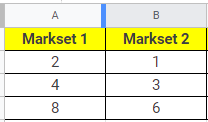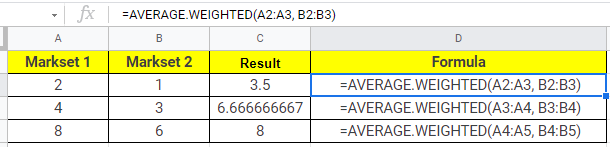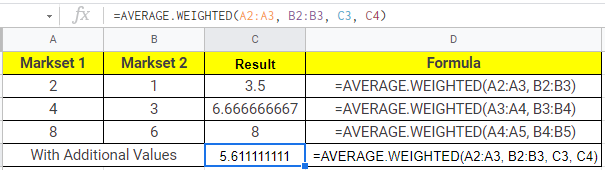## How To Calculate Weighted Average In Google Sheets (AVERAGE.WEIGHTED function)

AVERAGE.WEIGHTED function: When working with a large dataset in Google Sheets, calculating the average is a regular activity. The weighted arithmetic mean will provide a better representation of the data when the dataset is huge. In this article, let’s understand how to calculate the weighted average for the given dataset with the help of Google Sheet tips provided on this page. Read on to find out more.

## AVERAGE.WEIGHTED Function Syntax

The syntax of the average weighted function is:

• values: Values are the numbers that will be averaged. It might be a range of cells or just the values themselves.
• weights: Weights are the corresponding weights list to use. It may be a range of cells, or it could just be the weights themselves.  Weights are not allowed to be negative, although they can be zero. A positive weight must be present in at least one of the weights. If you are going to use a range of cells, make sure it has the same number of rows and columns as the values range.

### How to Calculate Weighted Average in Google Sheets?

Let’s understand how to calculate the weighted average in Google Sheets with the help of the following example dataset:Now let’s calculate the weighted average for the given numbers in Google Sheets by following the steps listed below:

• Step 1: Move the cell where you would like to draw the results using the weighted average function in Google Sheets.
• Step 2: Now enter the formula as “=AVERAGE.WEIGHTED(A1:A2, B1:B2)“. In our case, the weighted average formula is =AVERAGE.WEIGHTED(A2:A3, B2:B3), since we have considered row 1 as headers.
• Step 3: Press the “Enter” button. You will see the results.
• Step 4: Now drag the Fill Handle from the formula applied cell to other rows to apply the same formula to all other cells.### How to Calculate Weighted Average in Google Sheets using Additional Values?

Let us consider the same example which we have used in the above section. In addition to the above formula, let’s add the additional values.